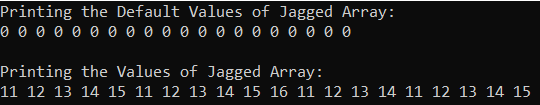# 2D Arrays in C#

## 2D Arrays in C# with Examples

In this article, I am going to discuss the 2D Arrays in C# with Examples. Please read our previous article before proceeding to this article where we discussed One-Dimensional Arrays in C# with Examples.

##### What is a Two-Dimensional Array in C#?

The arrays which store the elements in the form of rows and columns are called Two-Dimensional Array in C#. The two-dimensional array which is also called a multidimensional array is of two types in C#. They are as follows

1. Rectangular Array: The array whose rows and columns are equal is called a rectangular array
2. Jagged Array: The array whose rows and columns are not equal is called a jagged array
##### Rectangular 2D Arrays in C#:

A two-dimensional array is an array in which each element is referred to by two indexes. Element in the 2D array is stored in the matrix form. The first index shows the row of the matrix and the second index shows the column of the matrix.

Example: int[,] matrix = new int[3,3];

2D Array in Memory Representation is shown below. To access elements in the zeroth index we need to specify two indexes matrix.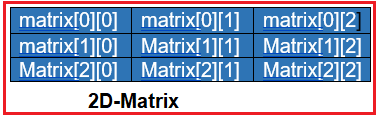##### How 2D Array is Created and Accessed in C#?

The method for creating a rectangular two-dimensional array is as follows:
int[,] A = new int[3,4];
If we created it like this, then the 2D array is created with 3 rows and 4 columns where the name of the array is A. For a better understanding, please have a look at the below diagram.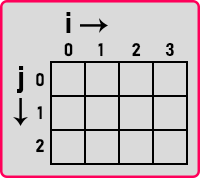Here, j represents the row number and i represents the column number. As we created the array with sizes 3 and 4, here you can see that the array is created with 3 rows and 4 columns. We can access any element with the row and column number as follows:
Console.WriteLine(A[1,2]);
This means the 2nd row and 3rd column as Array Indexed are 0-based indexes.

Note: Indexing starts from 0 onwards in the array. So, we have started the row and column from 0. This is how we can access any location. Addressing of 2d array is mapped the same as single dimension array.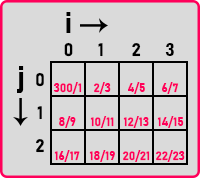Memory Locations are allocated continuously side by side. So basically, it will create a single dimension array of size 12, where the first four locations are used as the first rows, the second four locations are used as the second row and the rest four locations are used as the third row. So, in a computer the memory is represented as follows: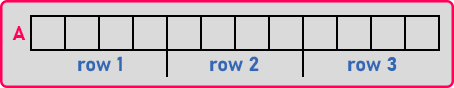But the compiler will allow us to access this single-dimension array as a 2D array. Next, let us see how to create and initialize a 2D array.

##### Initializing a 2Dimensional Array in C#:

Let us understand how to initialize a 2D Array with an example. Please have a look at the following statement which shows the declaration and initialization of a 2D Array.
int[,] A = {{2, 5, 9},{6, 9, 15}};
This is the declaration + initialization of a 2Dimensinal array in C#. Here 2,5,9 is the 1st row and 6,9,15 is the 2nd row. This is how they will be filled and we can access any element with the help of two indexes that is row number and column number. Now, the other way of initializing it is,
int[,] A = new int[2,3]
{
{2, 5, 9},{6, 9, 15}
};
Later part of this article, we will see how to initialize the 2D Array dynamically in C#. The following image shows the syntax of how we can initialize a 2D Rectangle Array in C#.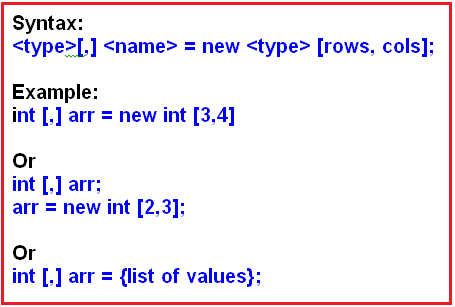Next, let us see how to access the elements of the 2-D array.

##### Accessing the Elements of the 2D array in C#:

For accessing all the elements of the rectangle 2D Array in C#, we require a nested for loop, one for loop for accessing the rows, and another for loop for accessing the columns. So, by using a nested for loop we can access 2Dimensional Array Elements. For a better understanding, please have a look at the below image.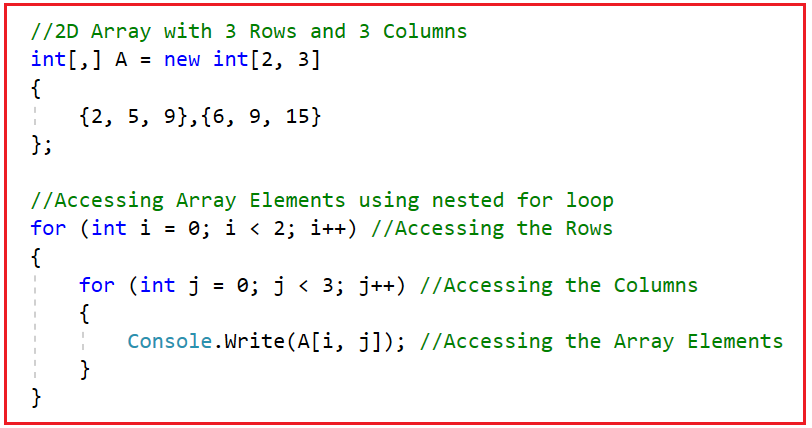##### Example to Understand 2D Array in C#:

Let us see an example for a better understanding of the rectangular 2D array in C#. In the below example, we are creating a two-dimensional integer array with 4 Rows and 5 Columns. Then we are printing the values of the 2D Array using a for each loop to see what default it stores. Then using nested for loop, we are assigning the values to the 2D Array as well as Printing the values of the 2D Array. In the below example, we are using the Array class GetLength method, when we pass 0, it will return the size of the Rows and when we pass 1, it will return the size of the columns.

```using System;
namespace TwoDimensionalArayDemo
{
class Program
{
static void Main(string[] args)
{
//Creating a 2D Array with 4 Rows and 5 Columns
int[,] RectangleArray = new int[4, 5];
int a = 0;

//Printing the values of 2D array using foreach loop
//It will print the default values as we are not assigning
//any values to the array
foreach (int i in RectangleArray)
{
Console.Write(i + " ");
}
Console.WriteLine("\n");

//Assigning values to the 2D array by using nested for loop
//arr.GetLength(0): Returns the size of the Row
//arr.GetLength(0): Returns the size of the Column
for (int i = 0; i < RectangleArray.GetLength(0); i++)
{
for (int j = 0; j < RectangleArray.GetLength(1); j++)
{
a += 5;
RectangleArray[i, j] = a;
}
}

//Printing the values of array by using nested for loop
//arr.GetLength(0): Returns the size of the Row
//arr.GetLength(0): Returns the size of the Column
for (int i = 0; i < RectangleArray.GetLength(0); i++)
{
for (int j = 0; j < RectangleArray.GetLength(1); j++)
{
Console.Write(RectangleArray[i, j] + " ");
}
}
}
}
}
```
###### Output: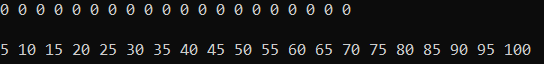In the above example, we assigned the two-dimensional array elements using nested for loop. It is also possible that we can assign the values to a two-dimensional array in C# at the time of its declaration.

##### 2D Array Declaration and Initialization at the Same Statement:

In the below example, we are assigning values to the two-dimensional array at the time of its declaration. Here, we do not need to specify the size as based on the argument values it will automatically choose the size. Here, it will create the array with the size of 3 Rows and 4 Columns. Then, we Printed the Array Elements using both ForEach Loop and Nested for Loop. In the nested for loop, the first for loop, loop variable i will point to the Rows, and in the second for loop, the loop variable I will point to the columns of the 2D Array.

```using System;
namespace TwoDimensionalArayDemo
{
class Program
{
static void Main(string[] args)
{
//Assigning the Array Elements at the time of declaration
//Row Size: 3
//Column Size: 4
int[,] NumbersArray = {{11,12,13,14},
{21,22,23,24},
{31,32,33,34}};

//Printing Array Elements using for each loop
Console.WriteLine("Printing Array Elements using ForEach loop");
foreach (int i in NumbersArray)
{
Console.Write(i + " ");
}

//Printing Array Elements using nested for each
Console.WriteLine("\n\nPrinting Array Elements using Nested For Loop");
for (int i = 0; i < NumbersArray.GetLength(0); i++)
{
for (int j = 0; j < NumbersArray.GetLength(1); j++)
{
Console.Write(NumbersArray[i, j] + " ");
}
}

}
}
}
```
###### Output: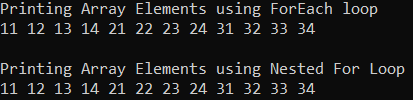##### Program to Add 2 Matrices using C#:
```using System;
namespace TwoDimensionalArayDemo
{
class Program
{
static void Main(string[] args)
{
Console.WriteLine("Enter rows and column of Matrices: ");

//Create 3 2D Arrays with the above size
int[,] Matrix1 = new int[Rows, Columns];
int[,] Matrix2 = new int[Rows, Columns];
int[,] ResultMatrix = new int[Rows, Columns];

//Enter Matrix 1 Elements
Console.WriteLine("\nEnter Elements of 1st Matrix:");
for (int i = 0; i < Rows; i++)
{
for (int j = 0; j < Columns; j++)
{
}
}

//Enter Matrix 2 Elements
Console.WriteLine("\nEnter Elements of 2nd Matrix:");
for (int i = 0; i < Rows; i++)
{
for (int j = 0; j < Columns; j++)
{
}
}

//Adding Both Matrix Elements and Store the Result in ResultMatrix
Console.WriteLine("\nSum of Both the Matrics:");
for (int i = 0; i < 2; i++)
{
for (int j = 0; j < 3; j++)
{
ResultMatrix[i,j] = Matrix1[i,j] + Matrix2[i,j];

Console.Write(\$"{ResultMatrix[i, j]} ");
}
Console.WriteLine();
}

}
}
}
```
###### Output: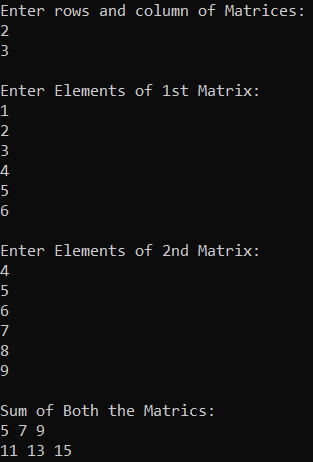##### Jagged Array in C#:

These are also two-dimensional arrays that will also store the data in the forms of rows and columns. But here in the jagged array, the column size will differ from row to row. That means if the first row contains 5 columns, then the second row may contain 4 columns while the third row may contain 10 columns. So, the point that you need to remember is if the column size varies from row to row then it is a jagged array. If the column size remains the same for all the rows, then it is a rectangular two-dimensional array.

The jagged array in C# is also called the array of arrays. This is because in the case of the jagged array each row is a single-dimensional array. So, a combination of multiple single-dimensional arrays with different column sizes forms a jagged array in C#.

Syntax:  <type> [][] <name> = new <type> [rows][];
Example:
int [][] arr = new int[];
//Or
int [][] arr = {list of values};

To declare a jagged array in C#, at the time of its declaration, you only need to specify the number of rows that you want in the array. For example
int [][] arr = new int[];

In the above array declaration, we are specifying that we want four rows in the array. Once you specify the number of rows that you want in the array, then you need to initialize each row with the number of columns by using a single-dimensional array as shown below.
arr = new int; // we want five columns in the first row
arr = new int; // we want six columns in the first row
arr = new int; // we want four columns in the first row
arr = new int; // we want five columns in the first row

##### Example to Understand Jagged Array in C#:

Jagged Arrays in C# are nothing but the combination of multiple 1D Arrays. In the below example, we created one jagged array with 4 Rows and then we initialize each row of the Jagged Array with different 1-Dimensional Arrays. Then we print the default values of jagged array using the nested for loop and then we initialize the jagged array using the nested for loop. And finally, once we initialized the jagged array, then we print the elements of a jagged array using a for loop and for each loop.

```using System;
namespace TwoDimensionalArayDemo
{
class Program
{
static void Main(string[] args)
{
//Creating An jagged array with four Rows
int[][] arr = new int[];

//Initializing each row with different column size
//Uisng one dimensional array
arr = new int;
arr = new int;
arr = new int;
arr = new int;

//Printing the values of Jagged array using nested for loop
//It will print the default values as we are not assigning any
//values to the array
//GetLength(0): Returns the Size of the Rows (4)
Console.WriteLine("Printing the Default Values of Jagged Array:");
for (int i = 0; i < arr.GetLength(0); i++)
{
//arr[i].Length: Returns the Length of Each Row
for (int j = 0; j < arr[i].Length; j++)
{
Console.Write(arr[i][j] + " ");
}
}

//Assigning values to the Jagged array by using nested for loop
for (int i = 0; i < arr.GetLength(0); i++)
{
int num = 10;
for (int j = 0; j < arr[i].Length; j++)
{
num++;
arr[i][j] = num;
}
}

//Printing the values of Jagged array by using foreach loop within for loop
Console.WriteLine("\n\nPrinting the Values of Jagged Array:");
for (int i = 0; i < arr.GetLength(0); i++)
{
foreach (int x in arr[i])
{
Console.Write(x + " ");
}
}

//You cannot simply use a foreach loop to Print the Values of a foreach loop
//foreach (var item in arr)
//{
//    Console.Write(item);
//}